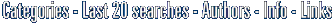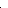# fMath Formula - GWT Widget fMath Formula - GWT Widget provide a simple way to display equations in GWT app. bestO in short: a - GWT Widget provide a simple way to display equations or mathematics formula on your GWT applications (Google Web Toolkit). It has more than 20000 symbols to display using MathML or in Larecent

Easy software similar to fMath Formula - GWT Widget, about:
widget and math editor or free mathml and cheap latex or the mathematics, good free or also components, image generation and equation or free algebraCategoriesfMath Formula - GWT Widget 1.5.1Click to enlarge

Description:
fMath Formula - GWT Widget provide a simple way to display equations or mathematics formula on your GWT applications (Google Web Toolkit). It has more than 20000 symbols to display using MathML or in LaTeX. The widget is free of charge to use and to distribute.

Type: Freeware
File size: 12209 Kb
Date: 02/18/2011
Homepage
Install support: Install Only
OS: WinVista, Unix, Linux, Mac OS X 10.5
System requirements: Web Browser
Language: English
Recent changes in this Major Update:
fMath Formula - GWT Widget provide a simple way to display equations or mathematics formula on your applications built with GWT (Google Web Toolkit). The Widget allow you to display the formula and to change the size, font, color and background.

Home and Education: Home and Education:Geometry and Mathematic Analysis

 FindGraphFindGraph is a graphing, curve-fitting, and digitizing tool for engineers, scientists and business. Discover the model that best describes your data.

 Visual CalculusVisual Calculus is an easy-to-use calculus grapher for Graphing limit, derivative function, integral, 3D vector, series, ode etc. Which can create 2D, 2.5D and 3D function graphs, animations and table graphs.

 Prime or notSearch, count, save and find primes then prove their primality.Using 4 tools:-The Primality Tester.-Mersenne numbers tester.-The Divisors finder.-The Search engine.-The convertor: Mersenne form-Fermat form-integer-The Splitter...

 Math-Flight 2Learning mathematics can be a challenge for anyone. Math Flight can help you master it with three fun activities to choose from! With lots of graphics and sound effects, your interest in learning math should never decline.

 Visual FractalWith this interesting fractal software, you can use Newton's method to solve a complex equation and show the fractal graph in the plot area.

 Visual ComplexVisual Complex is a graph software to create graph of complex function. 3D function graphs and 2D color maps can be created with this grapher.

 See above information and user's reviews about fMath Formula - GWT Widget fMath Formula - GWT Widget provide a simple way to display equations in GWT app. best See also other good software tools: Home and Education: Home and Education:Geometry and Mathematic AnalysisSearch Soft14: Search: the Web within this Web site